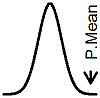P.Mean: What does it really mean to say that a mean of a large number of variables is approximately normal (created 2013-01-14). News: Sign up for "The Monthly Mean," the newsletter that dares to call itself average, www.pmean.com/news.Someone was looking at the Wikipedia page for the normal distribution and noted a comment that read "Normal distributions are extremely important in statistics, and are often used in the natural and social sciences for real-valued random variables whose distributions are not known. One reason for their popularity is the central limit theorem, which states that, under mild conditions, the mean of a large number of random variables independently drawn from the same distribution is distributed approximately normally, irrespective of the form of the original distribution." What does this mean exactly?

There are two independent issues here. First, what are these "mild conditions"? The conditions under which the Central Limit Theorem (CLT) hold are fairly well defined. You need to have independent and identically distributed random variables from a population distribution with a finite second moment. In practice, the finite second moment is usually a realistic thing to hope for. There are a few setting, particularly ratios where the denominator of the ratio has a fair chance of being very small, where a finite second moment would not apply. But the situations where it would not be reasonable to expect a finite second moment are relatively rare.

There are variations on the Central Limit Theorem that can mildly relax the assumptions about "independent" and about "identically distibuted," but the details here get very technical very fast. See the Wikipedia page on the CLT for an overview. I learned about all of this from a very good book by Robert Serfling back in graduate school, but that book is so old that I hesitate to mention it for fear of showing how old I am myself. For what it's worth, the deviations from independence and/or the deviations from identically distributed have to be bounded in the limit. That means that small deviations are fine, but large deviations might cause a problem.

There's a second issue, though. How large does a sample need to be in order for it to be safe for you to use the CLT? There is no uniform answer to this. If you look at the mathematical details (the Berry-Esseen theorem), it all depends on the third absolute moment of the distribution. Sometimes 10 is enough, sometimes 100 is not enough. It is pretty easy to show cases where the CLT holds, but you need thousands if not millions of cases before you get anything even close to a normal distribution. The sum of a bunch of gamma distributions, for example, is also a gamma distribution if they all have the same scale parameter. So pick a gamma distribution that is very far from normal and split it into however many pieces you want. The pieces of the gamma will be wildly skewed, with a shape parameter that is very close to zero. It is this extreme skewness in the individual gamma components that slows down the convergence.

These examples, though, are mostly only of interest to academic types, and the rule of 30 observations that is widely cited probably works well enough in practice.

The practical setting where you have to worry about whether 30 is large enough is when the underlying distribution tends to produce lots of outliers and when the underlying distribution is extremely skewed. Both of these will greatly increase the absolute third moment and will make convergence much slower. So those are the sorts of distributions where the CLT can only work its magic very slowly. It's worth noting, though, that often a log transformation can often insure very rapid convergence via the CLT in settings where the untransformed data has problems with skewness and/or outliers.This page was written by Steve Simon and is licensed under the Creative Commons Attribution 3.0 United States License. Need more information? I have a page with general help resources. You can also browse for pages similar to this one at Statistical Theory.